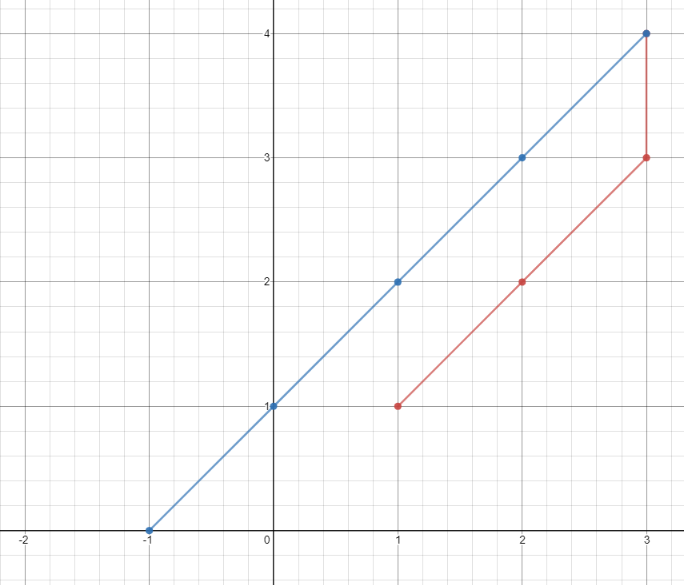##### Welcome to Subscribe On Youtube

Formatted question description: https://leetcode.ca/all/1266.html

# 1266. Minimum Time Visiting All Points (Easy)

On a plane there are n points with integer coordinates points[i] = [xi, yi]. Your task is to find the minimum time in seconds to visit all points.

You can move according to the next rules:

• In one second always you can either move vertically, horizontally by one unit or diagonally (it means to move one unit vertically and one unit horizontally in one second).
• You have to visit the points in the same order as they appear in the array.

Example 1:Input: points = [[1,1],[3,4],[-1,0]]
Output: 7
Explanation: One optimal path is [1,1] -> [2,2] -> [3,3] -> [3,4] -> [2,3] -> [1,2] -> [0,1] -> [-1,0]
Time from [1,1] to [3,4] = 3 seconds
Time from [3,4] to [-1,0] = 4 seconds
Total time = 7 seconds

Example 2:

Input: points = [[3,2],[-2,2]]
Output: 5


Constraints:

• points.length == n
• 1 <= n <= 100
• points[i].length == 2
• -1000 <= points[i], points[i] <= 1000

Related Topics:
Array, Geometry

## Solution 1.

// OJ: https://leetcode.com/problems/minimum-time-visiting-all-points/
// Time: O(N)
// Space: O(1)
class Solution {
int getDist(vector<int> &a, vector<int> &b) {
return max(abs(a - b), abs(a - b));
}
public:
int minTimeToVisitAllPoints(vector<vector<int>>& points) {
int ans = 0;
for (int i = 1; i < points.size(); ++i) {
ans += getDist(points[i - 1], points[i]);
}
return ans;
}
};

• class Solution {
public int minTimeToVisitAllPoints(int[][] points) {
int time = 0;
int length = points.length;
for (int i = 1; i < length; i++) {
int[] prevPoint = points[i - 1];
int[] curPoint = points[i];
int curTime = Math.max(Math.abs(curPoint - prevPoint), Math.abs(curPoint - prevPoint));
time += curTime;
}
return time;
}
}

• // OJ: https://leetcode.com/problems/minimum-time-visiting-all-points/
// Time: O(N)
// Space: O(1)
class Solution {
int getDist(vector<int> &a, vector<int> &b) {
return max(abs(a - b), abs(a - b));
}
public:
int minTimeToVisitAllPoints(vector<vector<int>>& points) {
int ans = 0;
for (int i = 1; i < points.size(); ++i) {
ans += getDist(points[i - 1], points[i]);
}
return ans;
}
};

• class Solution:
def minTimeToVisitAllPoints(self, points: List[List[int]]) -> int:
res = 0
x0, y0 = points, points
for x1, y1 in points[1:]:
res += max(abs(x0 - x1), abs(y0 - y1))
x0, y0 = x1, y1
return res

############

# 1266. Minimum Time Visiting All Points
# https://leetcode.com/problems/minimum-time-visiting-all-points/

class Solution:
def minTimeToVisitAllPoints(self, points: List[List[int]]) -> int:
res = 0
for (prevX,prevY), (currX,currY) in zip(points, points[1:]):
res += max(abs(prevX-currX), abs(prevY-currY))

return res